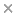BaseSpace
Correlation Engine 2.0Search sequence regions

filter terms:
Sizes of these terms reflect their relevance to your search.

By modeling the effects of predictor variables as a multiplicative function of regression parameters being invariant over categories, and category-specific scalar effects, the ordered stereotype logit model is a flexible regression model for ordinal response variables. In this article, we propose a generalized estimating equations (GEE) approach to estimate the ordered stereotype logit model for panel data based on working covariance matrices, which are not required to be correctly specified. A simulation study compares the performance of GEE estimators based on various working correlation matrices and working covariance matrices using local odds ratios. Estimation of the model is illustrated using a real-world dataset. The results from the simulation study suggest that GEE estimation of this model is feasible in medium-sized and large samples and that estimators based on local odds ratios as realized in this study tend to be less efficient compared with estimators based on a working correlation matrix. For low true correlations, the efficiency gains seem to be rather small and if the working covariance structure is too flexible, the corresponding estimator may even be less efficient compared with the GEE estimator assuming independence. Like for GEE estimators more generally, if the true correlations over time are high, then a working covariance structure which is close to the true structure can lead to considerable efficiency gains compared with assuming independence. © 2020 The Authors. Statistics in Medicine published by John Wiley & Sons, Ltd.

Citation

Martin Spiess, Daniel Fernández, Thuong Nguyen, Ivy Liu. Generalized estimating equations to estimate the ordered stereotype logit model for panel data. Statistics in medicine. 2020 Jun 30;39(14):1919-1940

PMID: 32227517

View Full Text